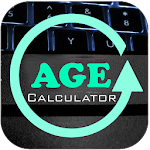ID: com.code1.agecalculator

## The description of Age Calculator

Age Calculator is calculate your age Year, month, weed, day, Hours an second.

## Age Calculator 1.0025 APK for Android 5.0+

 Version 1.0025 for Android 5.0+ Update on 2022-05-17 Installs 1.000.000++ File size 8.400.014 bytes Permissions view permissions What's new Fix some isue

### FAQs

• 11 Best Age Calculator Apps for Android and iOS. How Old Am I ? – Face Camera.Mental Age Calculator.Age Calculator Original by Rise Up Labs.Age Calculator by Android Code Play.Age Calculator Pro by ng-labs.Age Calculator by EasyAppDevTeam.Animal Years – Age Calculator.Ani – Real-Time Age Calculator.
• The date of birth refers to the date, the month and the year of a person's birth. It is used only in written English. Birthday refers to a day that is an exact number of years after the day when you were born. For example, it is your 22nd birthday and not birth date.
• Click the "Edit" link in the Basic Information section. Locate the Birthday section in your Google account's basic information. This is the birth date that you entered when you first registered for a Gmail account, and the date that displays on your Google profile.
• The method of calculating age involves the comparison of a person's date of birth with the date on which the age needs to be calculated. The date of birth is subtracted from the given date, which gives the age of the person. Age = Given date - Date of birth.
• Write down the current year and subtract the person's year of birth. For example, if the current year is 2011, subtract 1952 from 2011. You can either do this by hand with a pencil and paper, in your head or use a calculator. In this example, you should get an answer of 59.
• To calculate age using this method, first subtract the dates to calculate the number of days, then divide by 365.2422. Another common way to calculate the decimal number of years is to use the YEARFRAC function, however the result may not always be correct (e.g. 31-Jul-2012 to 30-Jul-2015).
• How to Calculate date of Birth | Birthday. To do this manually, you would need to know your exact current age and the date of the day when you're calculating it from. Then you can simple subtract the number of years from the current year, number of days and you will have found out your date of birth.